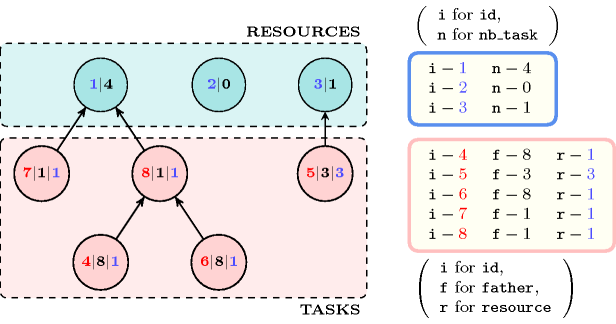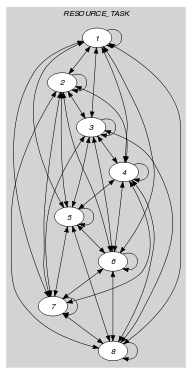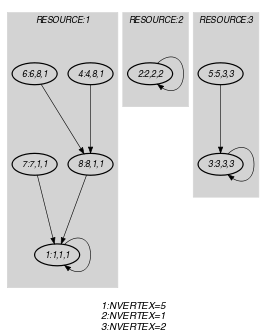## 5.405. tree_resource

Origin

Derived from $\mathrm{𝚝𝚛𝚎𝚎}$.

Constraint

$\mathrm{𝚝𝚛𝚎𝚎}_\mathrm{𝚛𝚎𝚜𝚘𝚞𝚛𝚌𝚎}\left(\mathrm{𝚁𝙴𝚂𝙾𝚄𝚁𝙲𝙴},\mathrm{𝚃𝙰𝚂𝙺}\right)$

Arguments
 $\mathrm{𝚁𝙴𝚂𝙾𝚄𝚁𝙲𝙴}$ $\mathrm{𝚌𝚘𝚕𝚕𝚎𝚌𝚝𝚒𝚘𝚗}\left(\mathrm{𝚒𝚍}-\mathrm{𝚒𝚗𝚝},\mathrm{𝚗𝚋}_\mathrm{𝚝𝚊𝚜𝚔}-\mathrm{𝚍𝚟𝚊𝚛}\right)$ $\mathrm{𝚃𝙰𝚂𝙺}$ $\mathrm{𝚌𝚘𝚕𝚕𝚎𝚌𝚝𝚒𝚘𝚗}\left(\mathrm{𝚒𝚍}-\mathrm{𝚒𝚗𝚝},\mathrm{𝚏𝚊𝚝𝚑𝚎𝚛}-\mathrm{𝚍𝚟𝚊𝚛},\mathrm{𝚛𝚎𝚜𝚘𝚞𝚛𝚌𝚎}-\mathrm{𝚍𝚟𝚊𝚛}\right)$
Restrictions
 $|\mathrm{𝚁𝙴𝚂𝙾𝚄𝚁𝙲𝙴}|>0$ $\mathrm{𝚛𝚎𝚚𝚞𝚒𝚛𝚎𝚍}$$\left(\mathrm{𝚁𝙴𝚂𝙾𝚄𝚁𝙲𝙴},\left[\mathrm{𝚒𝚍},\mathrm{𝚗𝚋}_\mathrm{𝚝𝚊𝚜𝚔}\right]\right)$ $\mathrm{𝚁𝙴𝚂𝙾𝚄𝚁𝙲𝙴}.\mathrm{𝚒𝚍}\ge 1$ $\mathrm{𝚁𝙴𝚂𝙾𝚄𝚁𝙲𝙴}.\mathrm{𝚒𝚍}\le |\mathrm{𝚁𝙴𝚂𝙾𝚄𝚁𝙲𝙴}|$ $\mathrm{𝚍𝚒𝚜𝚝𝚒𝚗𝚌𝚝}$$\left(\mathrm{𝚁𝙴𝚂𝙾𝚄𝚁𝙲𝙴},\mathrm{𝚒𝚍}\right)$ $\mathrm{𝚁𝙴𝚂𝙾𝚄𝚁𝙲𝙴}.\mathrm{𝚗𝚋}_\mathrm{𝚝𝚊𝚜𝚔}\ge 0$ $\mathrm{𝚁𝙴𝚂𝙾𝚄𝚁𝙲𝙴}.\mathrm{𝚗𝚋}_\mathrm{𝚝𝚊𝚜𝚔}\le |\mathrm{𝚃𝙰𝚂𝙺}|$ $\mathrm{𝚛𝚎𝚚𝚞𝚒𝚛𝚎𝚍}$$\left(\mathrm{𝚃𝙰𝚂𝙺},\left[\mathrm{𝚒𝚍},\mathrm{𝚏𝚊𝚝𝚑𝚎𝚛},\mathrm{𝚛𝚎𝚜𝚘𝚞𝚛𝚌𝚎}\right]\right)$ $\mathrm{𝚃𝙰𝚂𝙺}.\mathrm{𝚒𝚍}>|\mathrm{𝚁𝙴𝚂𝙾𝚄𝚁𝙲𝙴}|$ $\mathrm{𝚃𝙰𝚂𝙺}.\mathrm{𝚒𝚍}\le |\mathrm{𝚁𝙴𝚂𝙾𝚄𝚁𝙲𝙴}|+|\mathrm{𝚃𝙰𝚂𝙺}|$ $\mathrm{𝚍𝚒𝚜𝚝𝚒𝚗𝚌𝚝}$$\left(\mathrm{𝚃𝙰𝚂𝙺},\mathrm{𝚒𝚍}\right)$ $\mathrm{𝚃𝙰𝚂𝙺}.\mathrm{𝚏𝚊𝚝𝚑𝚎𝚛}\ge 1$ $\mathrm{𝚃𝙰𝚂𝙺}.\mathrm{𝚏𝚊𝚝𝚑𝚎𝚛}\le |\mathrm{𝚁𝙴𝚂𝙾𝚄𝚁𝙲𝙴}|+|\mathrm{𝚃𝙰𝚂𝙺}|$ $\mathrm{𝚃𝙰𝚂𝙺}.\mathrm{𝚛𝚎𝚜𝚘𝚞𝚛𝚌𝚎}\ge 1$ $\mathrm{𝚃𝙰𝚂𝙺}.\mathrm{𝚛𝚎𝚜𝚘𝚞𝚛𝚌𝚎}\le |\mathrm{𝚁𝙴𝚂𝙾𝚄𝚁𝙲𝙴}|$
Purpose

Cover a digraph $G$ in such a way that each vertex belongs to one distinct tree. Each tree is made up from one resource vertex and several task vertices. The resource vertices correspond to the roots of the different trees. For each resource a domain variable $\mathrm{𝚗𝚋}_\mathrm{𝚝𝚊𝚜𝚔}$ indicates how many task-vertices belong to the corresponding tree. For each task a domain variable $\mathrm{𝚛𝚎𝚜𝚘𝚞𝚛𝚌𝚎}$ gives the identifier of the resource that can handle the task.

Example
$\left(\begin{array}{c}〈\begin{array}{cc}\mathrm{𝚒𝚍}-1\hfill & \mathrm{𝚗𝚋}_\mathrm{𝚝𝚊𝚜𝚔}-4,\hfill \\ \mathrm{𝚒𝚍}-2\hfill & \mathrm{𝚗𝚋}_\mathrm{𝚝𝚊𝚜𝚔}-0,\hfill \\ \mathrm{𝚒𝚍}-3\hfill & \mathrm{𝚗𝚋}_\mathrm{𝚝𝚊𝚜𝚔}-1\hfill \end{array}〉,\hfill \\ 〈\begin{array}{ccc}\mathrm{𝚒𝚍}-4\hfill & \mathrm{𝚏𝚊𝚝𝚑𝚎𝚛}-8\hfill & \mathrm{𝚛𝚎𝚜𝚘𝚞𝚛𝚌𝚎}-1,\hfill \\ \mathrm{𝚒𝚍}-5\hfill & \mathrm{𝚏𝚊𝚝𝚑𝚎𝚛}-3\hfill & \mathrm{𝚛𝚎𝚜𝚘𝚞𝚛𝚌𝚎}-3,\hfill \\ \mathrm{𝚒𝚍}-6\hfill & \mathrm{𝚏𝚊𝚝𝚑𝚎𝚛}-8\hfill & \mathrm{𝚛𝚎𝚜𝚘𝚞𝚛𝚌𝚎}-1,\hfill \\ \mathrm{𝚒𝚍}-7\hfill & \mathrm{𝚏𝚊𝚝𝚑𝚎𝚛}-1\hfill & \mathrm{𝚛𝚎𝚜𝚘𝚞𝚛𝚌𝚎}-1,\hfill \\ \mathrm{𝚒𝚍}-8\hfill & \mathrm{𝚏𝚊𝚝𝚑𝚎𝚛}-1\hfill & \mathrm{𝚛𝚎𝚜𝚘𝚞𝚛𝚌𝚎}-1\hfill \end{array}〉\hfill \end{array}\right)$

The $\mathrm{𝚝𝚛𝚎𝚎}_\mathrm{𝚛𝚎𝚜𝚘𝚞𝚛𝚌𝚎}$ constraint holds since the graph associated with the items of the $\mathrm{𝚁𝙴𝚂𝙾𝚄𝚁𝙲𝙴}$ and the $\mathrm{𝚃𝙰𝚂𝙺}$ collections corresponds to 3 trees (i.e., $|\mathrm{𝚁𝙴𝚂𝙾𝚄𝚁𝙲𝙴}|=3$): each tree respectively involves the vertices $\left\{1,4,6,7,8\right\}$, $\left\{2\right\}$ and $\left\{3,5\right\}$. They are depicted by Figure 5.405.1, where resource and task vertices are respectively coloured in blue and pink.

##### Figure 5.405.1. The three trees corresponding to the Example slot; each resource vertex (in blue) contains the information $\mathrm{𝚒𝚍}|\mathrm{𝚗𝚋}_\mathrm{𝚝𝚊𝚜𝚔}$ where $\mathrm{𝚗𝚋}_\mathrm{𝚝𝚊𝚜𝚔}$ is the number of tasks in the tree, while each task vertex (in pink) contains the information $\mathrm{𝚒𝚍}|\mathrm{𝚏𝚊𝚝𝚑𝚎𝚛}|\mathrm{𝚛𝚎𝚜𝚘𝚞𝚛𝚌𝚎}$ where $\mathrm{𝚏𝚊𝚝𝚑𝚎𝚛}$ is the index of its father in the tree and $\mathrm{𝚛𝚎𝚜𝚘𝚞𝚛𝚌𝚎}$ is the index of the corresponding root task in the tree.Typical
 $|\mathrm{𝚁𝙴𝚂𝙾𝚄𝚁𝙲𝙴}|>0$ $|\mathrm{𝚃𝙰𝚂𝙺}|>|\mathrm{𝚁𝙴𝚂𝙾𝚄𝚁𝙲𝙴}|$
Symmetries
• Items of $\mathrm{𝚁𝙴𝚂𝙾𝚄𝚁𝙲𝙴}$ are permutable.

• Items of $\mathrm{𝚃𝙰𝚂𝙺}$ are permutable.

Reformulation

The $\mathrm{𝚝𝚛𝚎𝚎}_\mathrm{𝚛𝚎𝚜𝚘𝚞𝚛𝚌𝚎}$$\left(\mathrm{𝚁𝙴𝚂𝙾𝚄𝚁𝙲𝙴},\mathrm{𝚃𝙰𝚂𝙺}\right)$ constraint can be expressed in term of a conjunction of one $\mathrm{𝚝𝚛𝚎𝚎}$ constraint, $|\mathrm{𝚃𝙰𝚂𝙺}|$ $\mathrm{𝚎𝚕𝚎𝚖𝚎𝚗𝚝}$ constraints and one $\mathrm{𝚐𝚕𝚘𝚋𝚊𝚕}_\mathrm{𝚌𝚊𝚛𝚍𝚒𝚗𝚊𝚕𝚒𝚝𝚢}$ constraint:

With respect to the Example slot we get the following conjunction of constraints:

$\mathrm{𝚝𝚛𝚎𝚎}$$\left(3,〈\mathrm{𝚒𝚗𝚍𝚎𝚡}-1\mathrm{𝚜𝚞𝚌𝚌}-1,$

$\mathrm{𝚒𝚗𝚍𝚎𝚡}-2\mathrm{𝚜𝚞𝚌𝚌}-2,$

$\mathrm{𝚒𝚗𝚍𝚎𝚡}-3\mathrm{𝚜𝚞𝚌𝚌}-3,$

$\mathrm{𝚒𝚗𝚍𝚎𝚡}-4\mathrm{𝚜𝚞𝚌𝚌}-8,$

$\mathrm{𝚒𝚗𝚍𝚎𝚡}-5\mathrm{𝚜𝚞𝚌𝚌}-3,$

$\mathrm{𝚒𝚗𝚍𝚎𝚡}-6\mathrm{𝚜𝚞𝚌𝚌}-8,$

$\mathrm{𝚒𝚗𝚍𝚎𝚡}-7\mathrm{𝚜𝚞𝚌𝚌}-1,$

$\mathrm{𝚒𝚗𝚍𝚎𝚡}-8\mathrm{𝚜𝚞𝚌𝚌}-1〉\right)$,

$\mathrm{𝚎𝚕𝚎𝚖𝚎𝚗𝚝}$$\left(8,〈1,2,3,1,3,1,1,1〉,1\right)$,

$\mathrm{𝚎𝚕𝚎𝚖𝚎𝚗𝚝}$$\left(3,〈1,2,3,1,3,1,1,1〉,3\right)$,

$\mathrm{𝚎𝚕𝚎𝚖𝚎𝚗𝚝}$$\left(8,〈1,2,3,1,3,1,1,1〉,1\right)$,

$\mathrm{𝚎𝚕𝚎𝚖𝚎𝚗𝚝}$$\left(1,〈1,2,3,1,3,1,1,1〉,1\right)$,

$\mathrm{𝚎𝚕𝚎𝚖𝚎𝚗𝚝}$$\left(1,〈1,2,3,1,3,1,1,1〉,1\right)$,

$\mathrm{𝚐𝚕𝚘𝚋𝚊𝚕}_\mathrm{𝚌𝚊𝚛𝚍𝚒𝚗𝚊𝚕𝚒𝚝𝚢}$$\left(〈1,3,1,1,1〉,$

$〈\mathrm{𝚟𝚊𝚕}-1\mathrm{𝚗𝚘𝚌𝚌𝚞𝚛𝚛𝚎𝚗𝚌𝚎}-4,$

$\mathrm{𝚟𝚊𝚕}-2\mathrm{𝚗𝚘𝚌𝚌𝚞𝚛𝚛𝚎𝚗𝚌𝚎}-0,$

$\mathrm{𝚟𝚊𝚕}-3\mathrm{𝚗𝚘𝚌𝚌𝚞𝚛𝚛𝚎𝚗𝚌𝚎}-1〉\right)$.

Keywords
Derived Collection
$\mathrm{𝚌𝚘𝚕}\left(\begin{array}{c}\mathrm{𝚁𝙴𝚂𝙾𝚄𝚁𝙲𝙴}_\mathrm{𝚃𝙰𝚂𝙺}-\mathrm{𝚌𝚘𝚕𝚕𝚎𝚌𝚝𝚒𝚘𝚗}\left(\begin{array}{c}\mathrm{𝚒𝚗𝚍𝚎𝚡}-\mathrm{𝚒𝚗𝚝},\hfill \\ \mathrm{𝚜𝚞𝚌𝚌}-\mathrm{𝚍𝚟𝚊𝚛},\hfill \\ \mathrm{𝚗𝚊𝚖𝚎}-\mathrm{𝚍𝚟𝚊𝚛}\hfill \end{array}\right),\hfill \\ \left[\begin{array}{c}\mathrm{𝚒𝚝𝚎𝚖}\left(\begin{array}{c}\mathrm{𝚒𝚗𝚍𝚎𝚡}-\mathrm{𝚁𝙴𝚂𝙾𝚄𝚁𝙲𝙴}.\mathrm{𝚒𝚍},\hfill \\ \mathrm{𝚜𝚞𝚌𝚌}-\mathrm{𝚁𝙴𝚂𝙾𝚄𝚁𝙲𝙴}.\mathrm{𝚒𝚍},\hfill \\ \mathrm{𝚗𝚊𝚖𝚎}-\mathrm{𝚁𝙴𝚂𝙾𝚄𝚁𝙲𝙴}.\mathrm{𝚒𝚍}\hfill \end{array}\right),\hfill \\ \mathrm{𝚒𝚝𝚎𝚖}\left(\begin{array}{c}\mathrm{𝚒𝚗𝚍𝚎𝚡}-\mathrm{𝚃𝙰𝚂𝙺}.\mathrm{𝚒𝚍},\hfill \\ \mathrm{𝚜𝚞𝚌𝚌}-\mathrm{𝚃𝙰𝚂𝙺}.\mathrm{𝚏𝚊𝚝𝚑𝚎𝚛},\hfill \\ \mathrm{𝚗𝚊𝚖𝚎}-\mathrm{𝚃𝙰𝚂𝙺}.\mathrm{𝚛𝚎𝚜𝚘𝚞𝚛𝚌𝚎}\hfill \end{array}\right)\hfill \end{array}\right]\hfill \end{array}\right)$
Arc input(s)

$\mathrm{𝚁𝙴𝚂𝙾𝚄𝚁𝙲𝙴}_\mathrm{𝚃𝙰𝚂𝙺}$

Arc generator
$\mathrm{𝐶𝐿𝐼𝑄𝑈𝐸}$$↦\mathrm{𝚌𝚘𝚕𝚕𝚎𝚌𝚝𝚒𝚘𝚗}\left(\mathrm{𝚛𝚎𝚜𝚘𝚞𝚛𝚌𝚎}_\mathrm{𝚝𝚊𝚜𝚔}\mathtt{1},\mathrm{𝚛𝚎𝚜𝚘𝚞𝚛𝚌𝚎}_\mathrm{𝚝𝚊𝚜𝚔}\mathtt{2}\right)$

Arc arity
Arc constraint(s)
 $•\mathrm{𝚛𝚎𝚜𝚘𝚞𝚛𝚌𝚎}_\mathrm{𝚝𝚊𝚜𝚔}\mathtt{1}.\mathrm{𝚜𝚞𝚌𝚌}=\mathrm{𝚛𝚎𝚜𝚘𝚞𝚛𝚌𝚎}_\mathrm{𝚝𝚊𝚜𝚔}\mathtt{2}.\mathrm{𝚒𝚗𝚍𝚎𝚡}$ $•\mathrm{𝚛𝚎𝚜𝚘𝚞𝚛𝚌𝚎}_\mathrm{𝚝𝚊𝚜𝚔}\mathtt{1}.\mathrm{𝚗𝚊𝚖𝚎}=\mathrm{𝚛𝚎𝚜𝚘𝚞𝚛𝚌𝚎}_\mathrm{𝚝𝚊𝚜𝚔}\mathtt{2}.\mathrm{𝚗𝚊𝚖𝚎}$
Graph property(ies)
 $•$$\mathrm{𝐌𝐀𝐗}_\mathrm{𝐍𝐒𝐂𝐂}$$\le 1$ $•$$\mathrm{𝐍𝐂𝐂}$$=|\mathrm{𝚁𝙴𝚂𝙾𝚄𝚁𝙲𝙴}|$ $•$$\mathrm{𝐍𝐕𝐄𝐑𝐓𝐄𝐗}$$=|\mathrm{𝚁𝙴𝚂𝙾𝚄𝚁𝙲𝙴}|+|\mathrm{𝚃𝙰𝚂𝙺}|$

For all items of $\mathrm{𝚁𝙴𝚂𝙾𝚄𝚁𝙲𝙴}$:

Arc input(s)

$\mathrm{𝚁𝙴𝚂𝙾𝚄𝚁𝙲𝙴}_\mathrm{𝚃𝙰𝚂𝙺}$

Arc generator
$\mathrm{𝐶𝐿𝐼𝑄𝑈𝐸}$$↦\mathrm{𝚌𝚘𝚕𝚕𝚎𝚌𝚝𝚒𝚘𝚗}\left(\mathrm{𝚛𝚎𝚜𝚘𝚞𝚛𝚌𝚎}_\mathrm{𝚝𝚊𝚜𝚔}\mathtt{1},\mathrm{𝚛𝚎𝚜𝚘𝚞𝚛𝚌𝚎}_\mathrm{𝚝𝚊𝚜𝚔}\mathtt{2}\right)$

Arc arity
Arc constraint(s)
 $•\mathrm{𝚛𝚎𝚜𝚘𝚞𝚛𝚌𝚎}_\mathrm{𝚝𝚊𝚜𝚔}\mathtt{1}.\mathrm{𝚜𝚞𝚌𝚌}=\mathrm{𝚛𝚎𝚜𝚘𝚞𝚛𝚌𝚎}_\mathrm{𝚝𝚊𝚜𝚔}\mathtt{2}.\mathrm{𝚒𝚗𝚍𝚎𝚡}$ $•\mathrm{𝚛𝚎𝚜𝚘𝚞𝚛𝚌𝚎}_\mathrm{𝚝𝚊𝚜𝚔}\mathtt{1}.\mathrm{𝚗𝚊𝚖𝚎}=\mathrm{𝚛𝚎𝚜𝚘𝚞𝚛𝚌𝚎}_\mathrm{𝚝𝚊𝚜𝚔}\mathtt{2}.\mathrm{𝚗𝚊𝚖𝚎}$ $•\mathrm{𝚛𝚎𝚜𝚘𝚞𝚛𝚌𝚎}_\mathrm{𝚝𝚊𝚜𝚔}\mathtt{1}.\mathrm{𝚗𝚊𝚖𝚎}=\mathrm{𝚁𝙴𝚂𝙾𝚄𝚁𝙲𝙴}.\mathrm{𝚒𝚍}$
Graph property(ies)
$\mathrm{𝐍𝐕𝐄𝐑𝐓𝐄𝐗}$$=\mathrm{𝚁𝙴𝚂𝙾𝚄𝚁𝙲𝙴}.\mathrm{𝚗𝚋}_\mathrm{𝚝𝚊𝚜𝚔}+1$

Graph model

For the second graph constraint, part (A) of Figure 5.405.2 shows the initial graphs associated with resources 1, 2 and 3 of the Example slot. For the second graph constraint, part (B) of Figure 5.405.2 shows the corresponding final graphs associated with resources 1, 2 and 3. Since we use the $\mathrm{𝐍𝐕𝐄𝐑𝐓𝐄𝐗}$ graph property, the vertices of the final graphs are stressed in bold. To each resource corresponds a tree of respectively 4, 0 and 1 task-vertices.

##### Figure 5.405.2. Initial and final graph of the $\mathrm{𝚝𝚛𝚎𝚎}_\mathrm{𝚛𝚎𝚜𝚘𝚞𝚛𝚌𝚎}$ constraint(a) (b)
Signature

Since the initial graph of the first graph constraint contains $|\mathrm{𝚁𝙴𝚂𝙾𝚄𝚁𝙲𝙴}|+|\mathrm{𝚃𝙰𝚂𝙺}|$ vertices, the corresponding final graph cannot have more than $|\mathrm{𝚁𝙴𝚂𝙾𝚄𝚁𝙲𝙴}|+|\mathrm{𝚃𝙰𝚂𝙺}|$ vertices. Therefore we can rewrite the graph property $\mathrm{𝐍𝐕𝐄𝐑𝐓𝐄𝐗}=|\mathrm{𝚁𝙴𝚂𝙾𝚄𝚁𝙲𝙴}|+|\mathrm{𝚃𝙰𝚂𝙺}|$ to $\mathrm{𝐍𝐕𝐄𝐑𝐓𝐄𝐗}\ge |\mathrm{𝚁𝙴𝚂𝙾𝚄𝚁𝙲𝙴}|+|\mathrm{𝚃𝙰𝚂𝙺}|$ and simplify $\underline{\overline{\mathrm{𝐍𝐕𝐄𝐑𝐓𝐄𝐗}}}$ to $\overline{\mathrm{𝐍𝐕𝐄𝐑𝐓𝐄𝐗}}$.